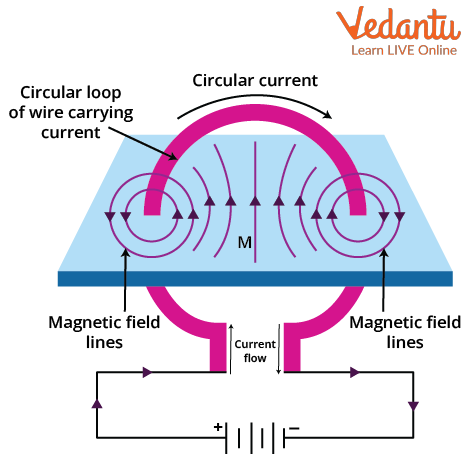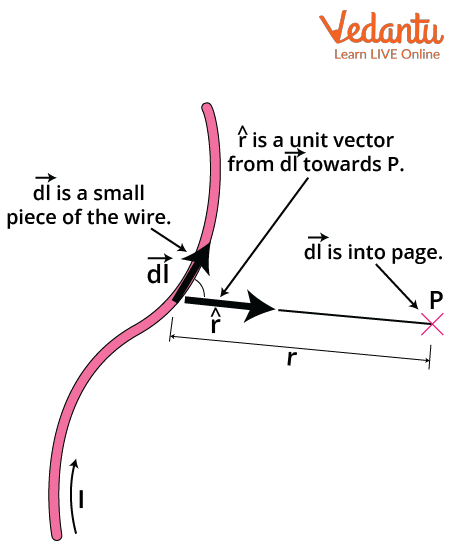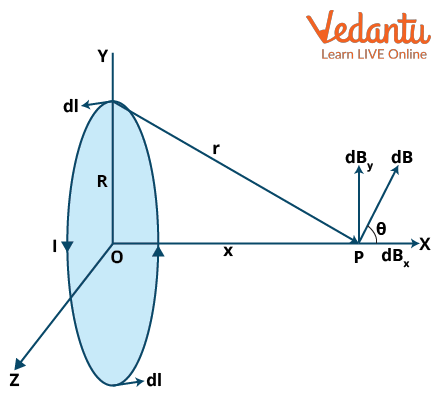Courses
Courses for Kids
Free study material
Free LIVE classes
More

# Magnetic Field on the Axis of a Circular Current Loop for JEELIVE
Join Vedantu’s FREE Mastercalss

## Magnetic Field Due to a Circular Loop

Let us take a magnetic compass and place it near a current-carrying circular loop. In this case, the needle present inside the magnetic compass experiences a torque and gets deflected to align along the direction of the magnetic field. The magnetic lines of force are almost parallel to one another near the centre, and form a circle away from the centre which implies that the magnetic field located at the centre of the circular coil is uniform.

The magnitude of the magnetic field increases with an increase in the number of turns, current or both. If the direction of the current is inverted, the needles present inside the magnetic field deflect in the opposite direction. This phenomenon introduces the theory of electromagnetism in Physics, which combines both electricity and magnetism.Magnetic lines of force around a circular loop

## Biot-Savart Law

How is the Biot -Savart law used in a current carrying conductor? The Biot-Savart law experimentally observes the magnitude of the magnetic field $\vec{dB}$ at a point P, of distance r from the small current element on a current-carrying conductor. Its magnitude is directly proportional to the strength of the current I, the magnitude of the length of the current element $\vec{dl}$, sine of the angle $\theta$ between the current element I$\vec{dl}$ and $\hat{r}$ and inversely proportional to the square of the distance r from the current element and the point.Biot-savart Law

$d B \propto \dfrac{I d l \sin \theta}{r^{2}}$

$d B=K \dfrac{I d l \sin \theta}{r^{2}}$

Here, K is the constant of proportionality and is equal to $\dfrac{\mu_{0}}{4 \pi}$.

This equation can be utilised to calculate only the magnetic field due to a small current element of length dl. So, by using the principle of superposition, the net magnetic field due to the entire conductor, which is made up of numerous current elements, can be calculated by summing up the magnetic field due to all current elements. Therefore, by integrating the magnetic field due to a current element we get:

$\vec{B}=\int \overrightarrow{d B}=\dfrac{\mu I}{4 \pi} \int \dfrac{\overrightarrow{d l} \times \hat{r}}{r^{2}}$

This integral is taken over the entire current distribution throughout the conductor.

## Derivation of Magnetic Field on the Axis of a Circular Current Loop

Let us consider a circular loop of radius R with current I flowing through it. Let P be the point and its distance from centre of the loop O be x. The magnetic field at point P is computed by taking two diametrically opposite line segments each of length $\vec{dl}$ at both ends of the wire, shown by the points from which the dl vector is taken in the diagram. The vector which is joining the current element at dl and P is $\hat{r}$.Magnetic field at a point P on the axis of a circular current loop

According to the Biot-Savart’s law, the magnetic induction at the point P due to the current element A is:

$\vec{d B}=\dfrac{\mu_{0}}{4 \pi} \dfrac{\vec{d l} \times \hat{r}}{r^{2}}$

The direction of $\vec{d B}$ is perpendicular to the current element I$\vec{d l}$and AP. Consequently, it is along the direction of PQ. The magnitude of magnetic field induction $\vec{d B}$ can be written as:

$d B=\dfrac{\mu_{0}}{4 \pi} \dfrac{I d l \sin \theta}{r^{2}}$

Here, $\theta$  is the angle between current element I$\vec{d l}$ and $\hat{r}$. Since its value is 90o, the magnitude of dB becomes:

$d B=\dfrac{\mu_{0}}{4 \pi} \dfrac{I d l}{r^{2}}$

Similarly, the magnitude of magnetic induction at P due to the current element at dl coming outside the page is the same as for current element at dl going inside the page due to their equal distances from the circular loop. But it has the opposite direction.

The magnetic field induction $\vec{d B}$ corresponding to each current element is resolved into two components $dB\cos{\theta}$ and $dB\sin{\theta}$ along the x-direction and y-direction respectively. The vertical components are equal and opposite in direction, hence they cancel each other whereas the horizontal components contribute to the total magnetic field. Hence, the net magnetic field B due to the entire circular loop at the point P is:

\begin{align} &\vec{B}=\int \overrightarrow{d B}=d B \cos \theta \hat{i} \\ &\vec{B}=\dfrac{\mu_{0}}{4 \pi} \int \dfrac{I d l}{r^{2}} \cos \theta \hat{i} \end{align}

By considering the triangle OAP as shown in the diagram, the value of  sin is

$\cos \theta=\dfrac{R}{\left(x^{2}+R^{2}\right)^{2}}$

Note that $r^{2}=R^{2}+x^{2}$

By substituting the value of $sin \,sin \,\alpha$ in the above equation, we get

$\vec{B}=\dfrac{\mu_{0}}{4 \pi} \dfrac{R}{\left(x^{2}+R^{2}\right)^{\dfrac{3}{2}}} \hat{i} \int d l$

Since it is a circular loop to calculate the net magnetic field at the point P, we integrate the line element from 0 to $2\pi R$

$\vec{B}=\dfrac{\mu_{0} I}{2} \dfrac{R^{2}}{\left(x^{2}+R^{2}\right)^{\dfrac{3}{2}}} \hat{i}$

If the circular loop contains N turns, then the magnetic field is given by:

$\vec{B}=\dfrac{\mu_{0} N I}{2} \dfrac{R^{2}}{\left(x^{2}+R^{2}\right)^{\dfrac{3}{2}}} \hat{i}$

The magnetic field is measured in $\dfrac{weber}{m^2}$.

The above derivation can be called the magnetic field on the axis of a circular current loop derivation. Also, from this formula, we can infer that a current carrying circular coil produces a uniform magnetic field.

## Special Cases for Magnetic Field Due to a Circular Conductor

1. The Magnetic Field at the Centre of the Circular Loop:

If we consider the magnetic field at the centre of the circular loop where the value of x is zero, then the net magnetic field at O is:

$\vec{B}=\dfrac{\mu_{0} N I}{2 R} \hat{i}$

This is the magnetic field at the centre of a circular coil formula.

2. Magnetic Field due to a Small Circular Loop:

If the circular loop is small  x>>R then the net magnetic field becomes:

$\vec{B}=\dfrac{\mu_{0} N I R^{2}}{x^{3}} \hat{i}$

## Conclusion

The magnetic field around a circular loop carrying current is uniform at the centre as the magnetic lines of the force are parallel to each other. The Biot-Savart law experimentally determines the magnetic field on a current carrying conductor. The magnetic field induction  at a point on the axis of a circular current loop is deduced as: $\vec{B}=\dfrac{\mu_{0} N I}{2} \dfrac{R^{2}}{\left(x^{2}+R^{2}\right)^{\dfrac{3}{2}}} \hat{i}$

Last updated date: 23rd Sep 2023
Total views: 132.6k
Views today: 1.32k

## FAQs on Magnetic Field on the Axis of a Circular Current Loop for JEE

1. What is an induced magnetic field?

When a magnetic field is induced, it indicates that the magnetic field is formed as a result of the primary field, i.e. the applied external field. When exposed to a changing magnetic field, all conductors display effective diamagnetism. Eddy currents are formed when electrons are subjected to the Lorentz force. Eddy currents then produce an induced magnetic field in the opposite direction of the applied field, which resists the conductor's motion. The strength of an induced magnetic field is given by Faraday’s law.

2. Where is the magnetic field stronger in a circular loop?

The magnetic field is stronger at the centre of the circular loop as the magnetic lines of force are very intense at the centre. They are also almost parallel to each other at the centre, which implies that the magnetic field is uniform at the centre. This has been experimentally shown and one can also calculate the field at the centre of the loop by using the formula for the magnetic field due to a circular loop at its axis.